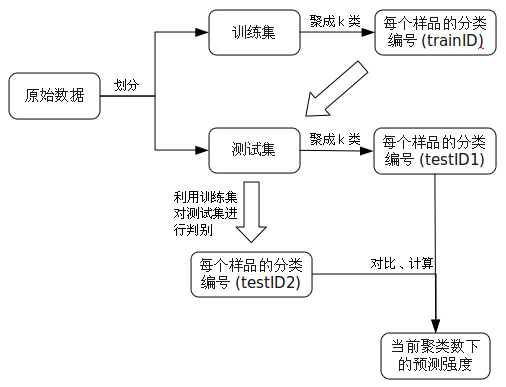## 预测强度及其在聚类分析中的应用

2009 年 11 月 08 日 | 分类于 学习中

### 二、预测强度的计算X1 X2 X3 X4 X5 X6 X7 X8 X9 X10 1 1 1 1 1 1 2 2 2 2

 X1 X2 X3 X4 X5 X6 X7 X8 X9 X10 1 1 2 1 1 2 2 2 2 1

 X1 X2 X3 X4 X5 X6 X1 1 0 1 1 0 X2 0 1 1 0 X3 0 0 1 X4 1 0 X5 0 X6

 X7 X8 X9 X10 X7 1 1 0 X8 1 0 X9 0 X10

### 四、一些思考

psk=function(train,test,k)
{
cl.train=kmeans(train,k)$cluster; cl.test=kmeans(test,k)$cluster;
n=table(cl.test);
library(MASS);
train=cbind(as.data.frame(train),cl=cl.train);
lda.model=lda(cl~.,data=train);
cl.test.pred=predict(lda.model,test)\$class;

if(is.vector(test)) test=as.matrix(test,ncol=1);
ps=numeric(k);
for(i in 1:k)
{
index=(1:dim(test))[cl.test==i];
pred=cl.test.pred[index];
ps[i]=(sum(outer(pred,pred,"=="))-n[i])/n[i]/(n[i]-1);
}
return(min(ps));
}